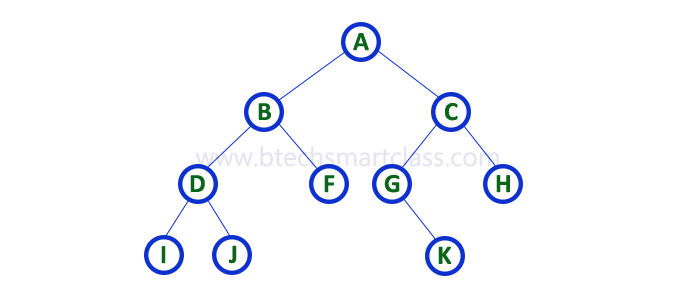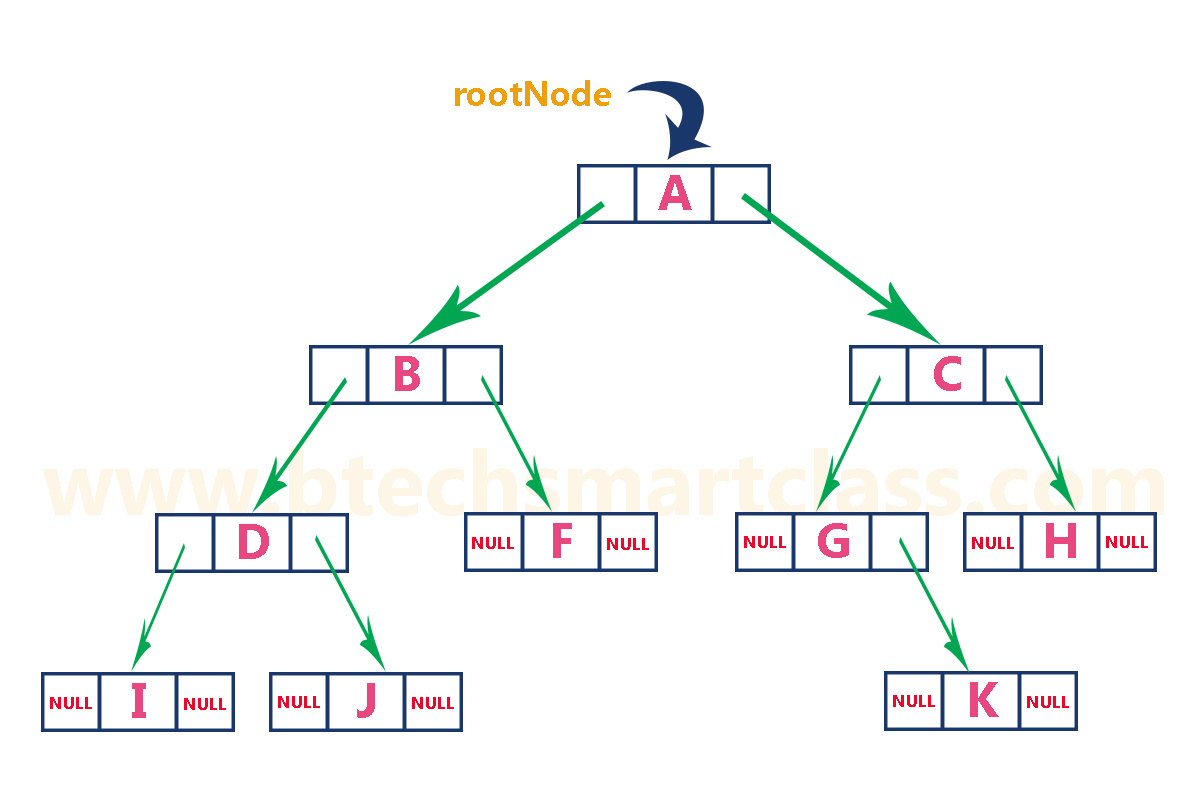The perfect place for easy learning...

×

# Binary Tree Representations

A binary tree data structure is represented using two methods. Those methods are as follows...

1. Array Representation

Consider the following binary tree...# 1. Array Representation of Binary Tree

In array representation of a binary tree, we use one-dimensional array (1-D Array) to represent a binary tree.
Consider the above example of a binary tree and it is represented as follows...To represent a binary tree of depth 'n' using array representation, we need one dimensional array with a maximum size of 2n + 1.

## 2. Linked List Representation of Binary Tree

We use a double linked list to represent a binary tree. In a double linked list, every node consists of three fields. First field for storing left child address, second for storing actual data and third for storing right child address.
In this linked list representation, a node has the following structure...The above example of the binary tree represented using Linked list representation is shown as follows...